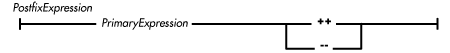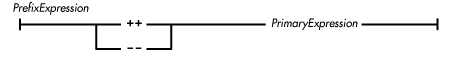start page | rating of books | rating of authors | reviews | copyrights

### 4.3 Increment/Decrement Operators

The ++ operator is used to increment the contents of a variable or an array element by one, while the - - operator is used to decrement such a value by one. The operand of ++ or - - must evaluate to a variable or an array element; it cannot be an expression that produces a pure value. For example, the following operations succeed because the operand of the ++ operator produces a variable:

```int g = 0;
g++;
```

However, the following uses of ++ generate error messages:

```final int h = 23;
h++;
5++;
```

The expression h++ produces an error because h is declared final, which means that its value cannot be changed. The expression 5++ generates an error message because 5 is a literal value, not a variable.

The increment and decrement operators can be used in both postfix expressions (e.g., i++ or i- -) and in prefix expressions (e.g., ++i or - -i). Although both types of expression have the same side effect of incrementing or decrementing a variable, they differ in the values that they produce. A postfix expression produces a pure value that is the value of the variable before it is incremented or decremented, while a prefix expression produces a pure value that is the value of the variable after it has been incremented or decremented. For example, consider the following code fragment:

```int i = 3, j = 3;
System.out.println( "i++ produces " + i++);
System.out.println( "++j produces " + ++j);
```

The above code fragment produces the following output:

```i++ produces 3
++j produces 4
```

After the code fragment has been evaluated, both i and j have the value 4.

In essence, what you need to remember is that a prefix expression performs its increment or decrement before producing a value, while a postfix expression performs its increment or decrement after producing a value.

### Postfix Increment/Decrement Operators

A postfix increment/decrement expression is a primary expression that may be followed by either a ++ or a - -:The postfix increment and decrement operators are equal in precedence and are effectively non-associative.

If a postfix expression includes a ++ or - -, the primary expression must produce a variable or an array element of an arithmetic type. The postfix increment operator (++) has the side effect of incrementing the contents of the variable or array element by one. The postfix decrement operator (- -) has the side effect of decrementing the contents of the variable or array element by one.

The data type of the value produced by a postfix increment/decrement operator is the same as the data type of the variable or array element produced by the primary expression. A postfix increment/decrement operator produces the original pure value stored in the variable or array element before it is incremented or decremented.

The following is an example of using a postfix decrement operator:

```char j = '\u0100';
while (j-- > 0)        // call doit for char values
doit(j);           // '\u00ff' through '\u0000'
```

This example works because Java treats char as an arithmetic data type.

### Prefix Increment/Decrement Operators

A prefix increment/decrement expression is a primary expression that may be preceded by either a ++ or a - -:The prefix increment and decrement operators are equal in precedence and are effectively non-associative.

If a prefix expression includes a ++ or - -, the primary expression must produce a variable or an array element of an arithmetic type. The prefix increment operator (++) has the side effect of incrementing the contents of the variable or array element by one. The prefix decrement operator (- -) has the side effect of decrementing the contents of the variable or array element by one.

The data type of the value produced by a prefix increment/decrement operator is the same as the data type of the variable or array element produced by the primary expression. A prefix increment/decrement operator produces the pure value stored in the variable or array element after it has been incremented or decremented.

Here's an example of using a prefix increment operator:

```void foo(int a[]) {
int j = -1;
while (++j < a.length)    // call doit for each element
doit(a[j]);              // of a
}
```